Example Questions

1 2 3 4 6 Next →

Example Question #12 : How To Find Decimal Fractions

Convert the mixed fraction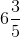to a decimal number.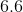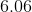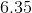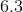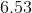Explanation:

Since the starting number is a mixed number fraction, the decimal number must also have a value in the ones place. Since the denominator in the fraction has a value that is a factor of, both the numerator and denominator can be multiplied by a factor ofto formtenths. (Note, the first decimal place value is the tenths place).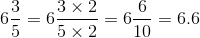Example Question #13 : How To Find Decimal Fractions

A retailer can only order wristbands in bulk cases. Each case has 300 wristbands and costs $1172. Quantity A: The number of wristbands that can be bought for$10547.

Quantity B: The number of wristbands that can be bought for $10560. Possible Answers: Quantity A is greater. The relationship cannot be determined. Quantity B is greater. The two quantities are equal. Correct answer: Quantity B is greater. Explanation: The key to this problem is to realize that the store cannot buy partial crates of wristbands--it's all or nothing. Calculate how many crates can be bought with each sum of money by dividing the sum by the price of a crate. Quantity A: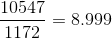Although oh so close, only eight crates can be bought with this sum of money. Don't round up! Quantity B: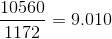There's just enough to buy nine crates. Quantity B is greater. Example Question #1 : How To Find The Fractional Equivalent Of A Decimal A tub of food containspounds of vegetables,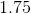pounds of lard, andpounds of sausage. What is its total weight as an improper fraction? Possible Answers: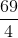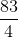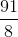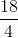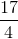Correct answer:Explanation: To begin with, it is easiest just to add these decimals together using your calculator: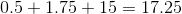Now, this is the same thing as: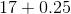We can rewrite this: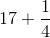To find this, you need to give the two numbers a common denominator: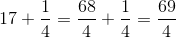This is your answer. Example Question #2 : How To Find The Fractional Equivalent Of A Decimal What is the fractional equivalent of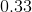? Possible Answers: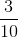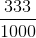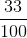Correct answer:Explanation: In decimal formis said 33 hundredths. This is equal to. This fraction cannot be reduced any further therefore it is in its final answer form. Example Question #3 : How To Find The Fractional Equivalent Of A Decimal Choose the answer which best converts the following decimal into a fraction, ensure that you simplify your answer: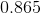Possible Answers: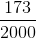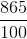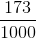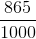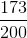Correct answer:Explanation: To convert a decimal into a fraction, simply put the digits of the decimal in the numerator of a fraction, then one followed by a number of zeroes equal to the number of digits in the decimal as your denomator. In this case:Then, you can simplify, as both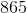and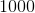are divisible by:Example Question #1 : How To Find The Fractional Equivalent Of A Decimal Simplify the following expression: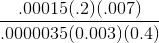Possible Answers: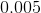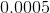Correct answer:Explanation: It can help to begin by writing the expression using scientific notation: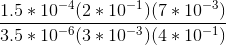Now remove common divisors from the numerator and denominator: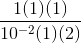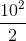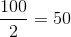Example Question #1 : Decimals If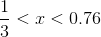can equal which of the following? Possible Answers: None of the other answer choices are correct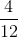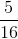Correct answer:Explanation: Convert all of the fractions to decimals. Thus, x is contained within the range of 0.33 < x < 0.76. The answers choices become 1/4 = 0.25, 4/12 = 0.33, 2/5 = 0.4, and 5/16 = 0.3125, respectively. Therefore, the only answer which is within the desired range is 2/5. Example Question #1 : How To Find The Decimal Equivalent Of A Fraction Vega only purchases gardening claws in bulk cases. Each case is 150 claws for$2415.17.

Quantity A: The number of claws Vega can purchase with $41,082.05 Quantity B: The number of claws Vega can purchase with$43,473.05

Quantity B is greater.

Quantity A is greater.

The two quantities are equal.

The relationship cannot be determined.

The two quantities are equal.

Explanation:

Looking at the differences in prices, one might guess that Quantity B is the answer. Beware the easy answer; always check.

Instead, calculating how many cases of claws Vega can buy, keeping in mind that he cannot purchase part of a case. Do not round up!

Quantity A: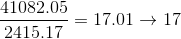Quantity B: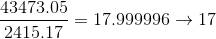In either case, he can buy no more than seventeen cases. The two quantities are equal.

Example Question #2 : How To Find The Decimal Equivalent Of A Fraction

Isley only buys seeds for her gardening store in bulk cases, with each case containingpackets of seeds and costing $343.25. Quantity A: The number of seeds Isley can purchase with$3837.54

Quantity B: The number of seeds Isley can purchase with $3871.86 Possible Answers: The relationship cannot be determined. Quantity A is greater. Quantity B is greater. The two quantities are equal. Correct answer: The two quantities are equal. Explanation: Looking at how close the dollar values are for A and B, it's tempting to think the GRE is being tricky, and that B will allow for the purchase of more cases of seeds. Do not assume; check to see whether or not this is the situation. Note that a portion of a case cannot be purchased; round down to the nearest integer when calculating how many cases may be bought. Since each case is$343.25

Quantity A: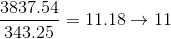Quantity B: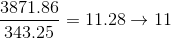The two quantities are equal.

Example Question #1 : How To Find The Decimal Equivalent Of A Fraction

Choose the answer which best expresses the following fraction in decimal form (round to the nearest thousandth):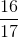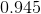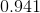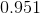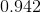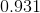Explanation:

To convert a fraction into a decimal, you simply need to divide the numerator by the denominator.  When the problem prompts you to round to the nearest thousandth, that means that you round to the third decimal place (i.e. go to the third decimal place, if the fourth is four or lower, you can leave the third decimal place digit alone, if the fourth is five or higher, raise the third decimal place digit by one).

When you divide 16 by 17 you will need to add a zero and a decimal place to 16 making it 160. From here we see that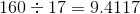but since we added a decimal place to 16 to create 160 we now need to move the decimal place of the answer one unit to the left.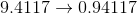1 2 3 4 6 Next →

Tired of practice problems?

Try live online GRE prep today.